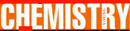# Density - Research Article from World of Chemistry

This encyclopedia article consists of approximately 5 pages of information about Density.
 This section contains 1,242 words (approx. 5 pages at 300 words per page) View a FREE sampleDensity is a measure of how much mass a substance has in comparison to its size. Density is defined as the ratio of mass to the volume of a particular substance. The mass of a material is directly proportional to the volume. If mass versus volume for a particular substance were plotted on a graph the result would be a straight line. The slope of this line would be the density of the substance. When calculating the density of a sample, the mass and the volume of the sample are determined and the mass of the sample is then divided by the volume. The Système International d'Unités (S.I.) unit for density measurements is kg/m3, although it is often expressed in g/cm3 or g/mL.

Conceptually, density can be described as how close together the molecules in a substance are packed. Every...

(read more)

 This section contains 1,242 words (approx. 5 pages at 300 words per page) View a FREE sampleMore summaries and resources for teaching or studying Density.
CopyrightsDensity from Gale. ©2005-2006 Thomson Gale, a part of the Thomson Corporation. All rights reserved.